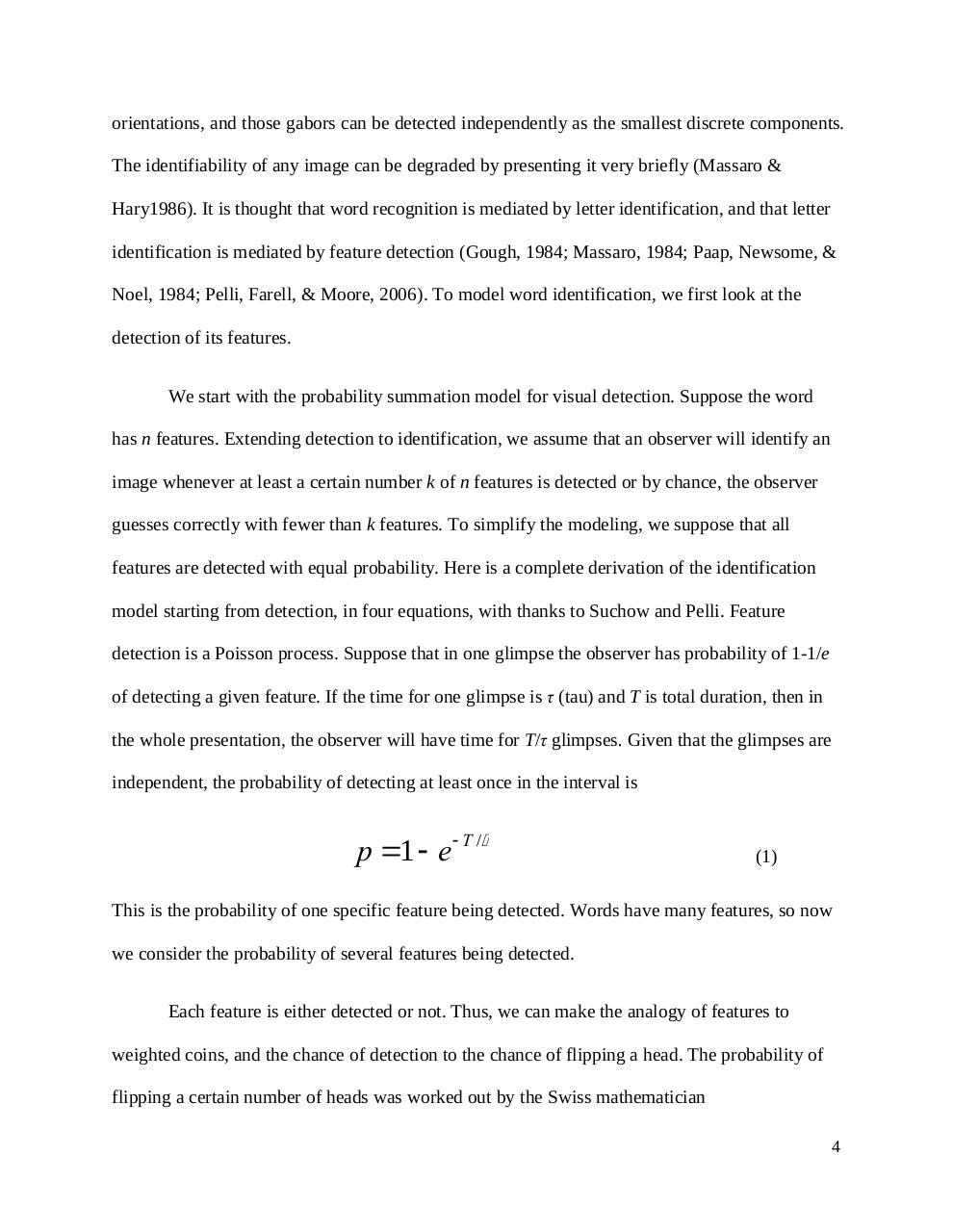# Quantifying Visual Feature Detection in Word Identification.pdfPage 1 2 3 45621

#### Text preview

orientations, and those gabors can be detected independently as the smallest discrete components.
The identifiability of any image can be degraded by presenting it very briefly (Massaro &amp;
Hary1986). It is thought that word recognition is mediated by letter identification, and that letter
identification is mediated by feature detection (Gough, 1984; Massaro, 1984; Paap, Newsome, &amp;
Noel, 1984; Pelli, Farell, &amp; Moore, 2006). To model word identification, we first look at the
detection of its features.
We start with the probability summation model for visual detection. Suppose the word
has n features. Extending detection to identification, we assume that an observer will identify an
image whenever at least a certain number k of n features is detected or by chance, the observer
guesses correctly with fewer than k features. To simplify the modeling, we suppose that all
features are detected with equal probability. Here is a complete derivation of the identification
model starting from detection, in four equations, with thanks to Suchow and Pelli. Feature
detection is a Poisson process. Suppose that in one glimpse the observer has probability of 1-1/e
of detecting a given feature. If the time for one glimpse is τ (tau) and T is total duration, then in
the whole presentation, the observer will have time for T/τ glimpses. Given that the glimpses are
independent, the probability of detecting at least once in the interval is

p =1 - e -T /t

(1)

This is the probability of one specific feature being detected. Words have many features, so now
we consider the probability of several features being detected.
Each feature is either detected or not. Thus, we can make the analogy of features to
weighted coins, and the chance of detection to the chance of flipping a head. The probability of
flipping a certain number of heads was worked out by the Swiss mathematician
4